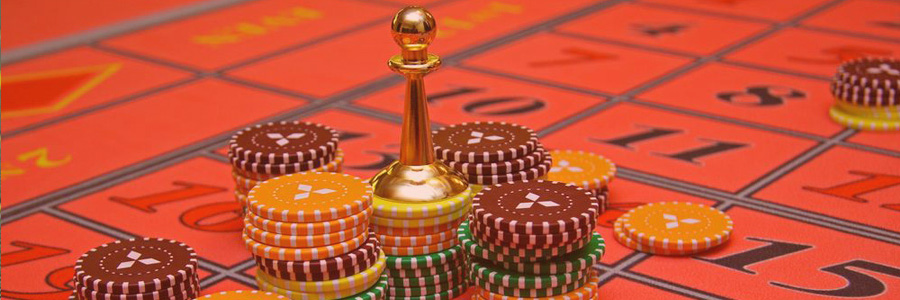# The Whittacker betting systemThis particolar betting system is mainly applied in roulette matches, especially in simple chance matches and during losing turns.

This betting system develops more slowly than the classical one. Indeed, you need more than 1 winning turn in order to balance the previous losing turns.

## Principle of the Whittacker betting system

The Whittacker game tactic allows you calculate the amount of your bets according to the previous bets.

There are several variation of this technique, but one is definitely the most appreciated so here you can see how it functions.

In case you are losing, you can put together the amounts of the two previous bets in creating the next one. On the other hand, if the result of the gains is positive, you will then bet a unit.

Another variation of this betting system consists in calculating the amount of the new bet, basing on the amounts of the three previous ones.

We need to take into consideration the limits of this technique for popular games: the bets tend to be done quite rapidly, has with any betting system.

It is really important therefore to fix a maximum amount and respect it.

## Example of how to apply the Whittacker betting system

First, we consider the man variation of this betting system, based on the total amount of the two previous bets.

1. For the first turn, the player bets a unit and s/he loses.
2. Thus, s/he bets an additional unit for the second turn and so two units. S/he loses.
3. When the third turn comes, the player applies the Whittacker betting system, betting 2+1=3 units. S/he loses.
4. For the fourth turn, s/he bets 2+3=5 units and s/he loses.
5. The fifth turn comes, and the player bets 3+5=8 units. S/he wins, with a result of 8-5-3-2= -2 units (a loss of two units).
6. For the sixth turn, s/he bets 8+5 = 13 units. S/he wins with a result of 13-2=11 (a gain of 11 units). For the next turn, the player will start betting with one unit.

Thus, two winning turns have been enough to balance the losses and win.

Do not forget to fix a maximum amount to bet in order to avoid to make too many bets to fast and also to be prudent.